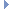OpenMM
HarmonicAngleForce Class Reference

This class implements an interaction between groups of three particles that varies harmonically with the angle between them. More...Inheritance diagram for HarmonicAngleForce:

List of all members.

## Public Member Functions

def getNumAngles
getNumAngles(self) -> int
addAngle(self, particle1, particle2, particle3, angle, k) -> int
def getAngleParameters
Get the force field parameters for an angle term.
def setAngleParameters
Set the force field parameters for an angle term.
def updateParametersInContext
Update the per-angle parameters in a Context to match those stored in this Force object.
def usesPeriodicBoundaryConditions
usesPeriodicBoundaryConditions(self) -> bool
def __init__
__init__(self) -> HarmonicAngleForce __init__(self, other) -> HarmonicAngleForce

this

## Detailed Description

This class implements an interaction between groups of three particles that varies harmonically with the angle between them.

To use it, create a HarmonicAngleForce object then call addAngle() once for each angle. After an angle has been added, you can modify its force field parameters by calling setAngleParameters(). This will have no effect on Contexts that already exist unless you call updateParametersInContext().

## Constructor & Destructor Documentation

 def __init__ ( self, args )

__init__(self) -> HarmonicAngleForce __init__(self, other) -> HarmonicAngleForce

Create a HarmonicAngleForce.

## Member Function Documentation

 def addAngle ( self, particle1, particle2, particle3, angle, k )

addAngle(self, particle1, particle2, particle3, angle, k) -> int

Add an angle term to the force field.

Parameters:
 particle1 (int) the index of the first particle forming the angle particle2 (int) the index of the second particle forming the angle particle3 (int) the index of the third particle forming the angle angle (double) the equilibrium angle, measured in radians k (double) the harmonic force constant for the angle, measured in kJ/mol/radian^2
Returns:
(int) the index of the angle that was added
 def getAngleParameters ( self, index )

Get the force field parameters for an angle term.

Parameters:
 index (int) the index of the angle for which to get parameters
Returns:
(int) the index of the first particle forming the angle
(int) the index of the second particle forming the angle
(int) the index of the third particle forming the angle
(double) the equilibrium angle, measured in radians
(double) the harmonic force constant for the angle, measured in kJ/mol/radian^2
 def getNumAngles ( self )

getNumAngles(self) -> int

Get the number of harmonic bond angle terms in the potential function

 def setAngleParameters ( self, index, particle1, particle2, particle3, angle, k )

Set the force field parameters for an angle term.

Parameters:
 index (int) the index of the angle for which to set parameters particle1 (int) the index of the first particle forming the angle particle2 (int) the index of the second particle forming the angle particle3 (int) the index of the third particle forming the angle angle (double) the equilibrium angle, measured in radians k (double) the harmonic force constant for the angle, measured in kJ/mol/radian^2
 def updateParametersInContext ( self, context )

Update the per-angle parameters in a Context to match those stored in this Force object.

This method provides an efficient method to update certain parameters in an existing Context without needing to reinitialize it. Simply call setAngleParameters() to modify this object's parameters, then call updateParametersInContext() to copy them over to the Context.

The only information this method updates is the values of per-angle parameters. The set of particles involved in a angle cannot be changed, nor can new angles be added.

 def usesPeriodicBoundaryConditions ( self )

usesPeriodicBoundaryConditions(self) -> bool

Returns whether or not this force makes use of periodic boundary conditions.

Returns:
(bool) true if force uses PBC and false otherwise

Reimplemented from Force.

## Member Data Documentation

Reimplemented from Force.

The documentation for this class was generated from the following file:
• openmm.py